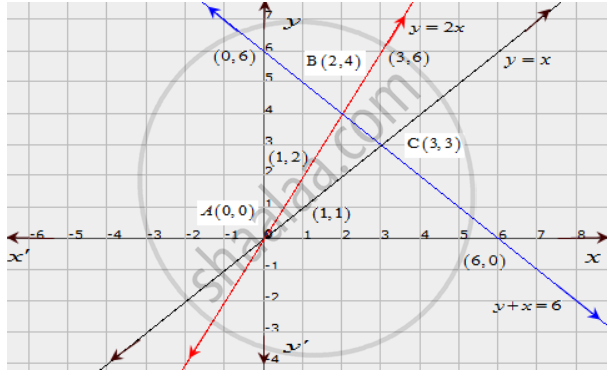Share

# Determine Graphically the Coordinates of the Vertices of a Triangle, the Equations of Whose Sides Are : Y = X, Y = 2x And Y + X = 6 - CBSE Class 10 - Mathematics

ConceptGraphical Method of Solution of a Pair of Linear Equations

#### Question

Determine graphically the coordinates of the vertices of a triangle, the equations of whose sides are :

y = x, y = 2x and y + x = 6

#### Solution

The given equations are

y = x  ........(i)

y = 2x .......(ii)

y + x = 6 ......(iii)

The two points satisfying (i) can be listed in a table as,

 x 0 1 y 0 1

The two points satisfying (ii) can be listed in a table as,

 x 1 3 y 2 6

The two points satisfying (iii) can be listed in a table as,

 x 0 6 y 6 6

Now, the graph of equations (i), (ii) and (iii) can be drawn as,It is seen that the coordinates of the vertices of the obtained triangle are A(0,0), B(2,4), C(3,3)

Is there an error in this question or solution?

#### APPEARS IN

Solution Determine Graphically the Coordinates of the Vertices of a Triangle, the Equations of Whose Sides Are : Y = X, Y = 2x And Y + X = 6 Concept: Graphical Method of Solution of a Pair of Linear Equations.
S